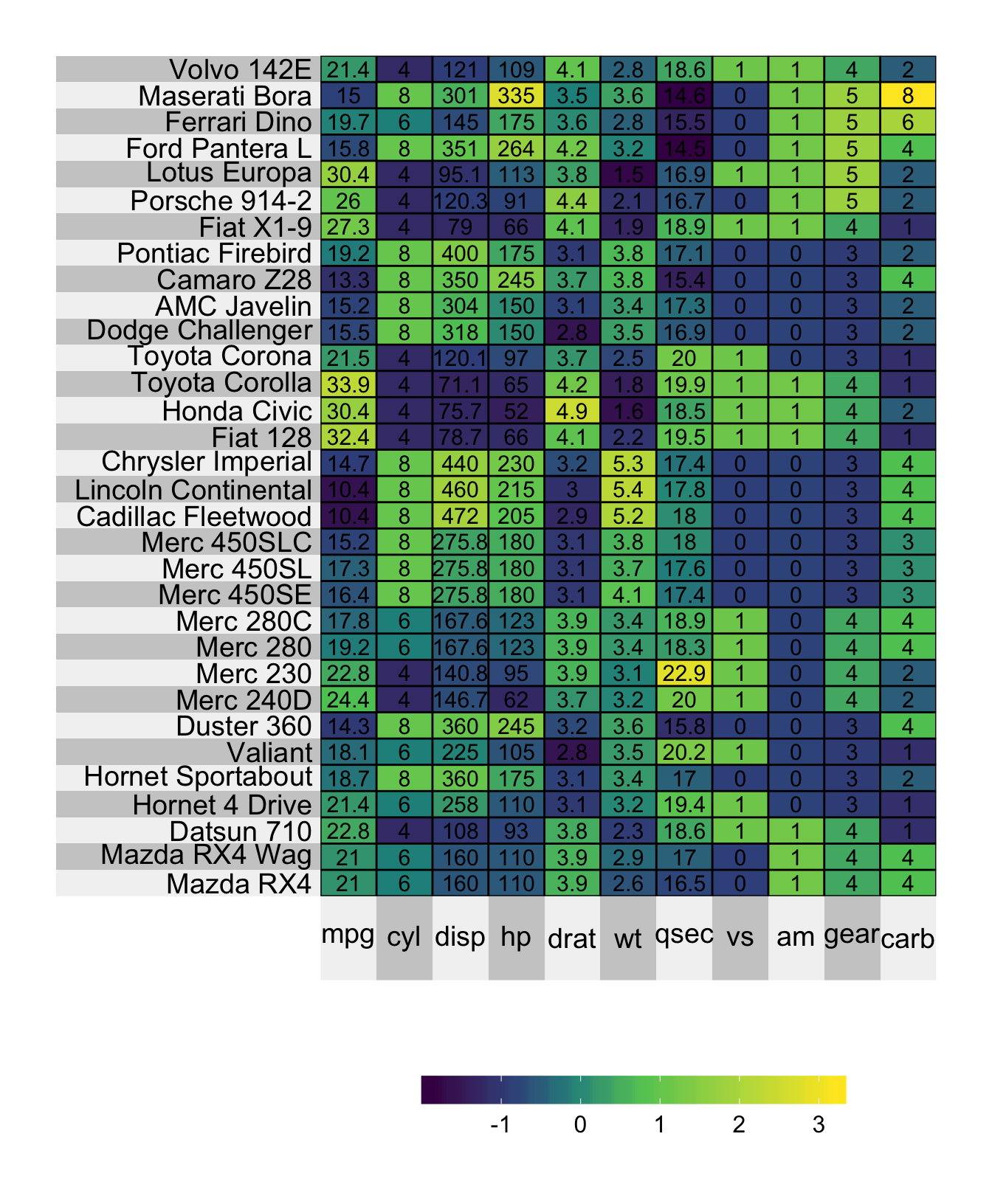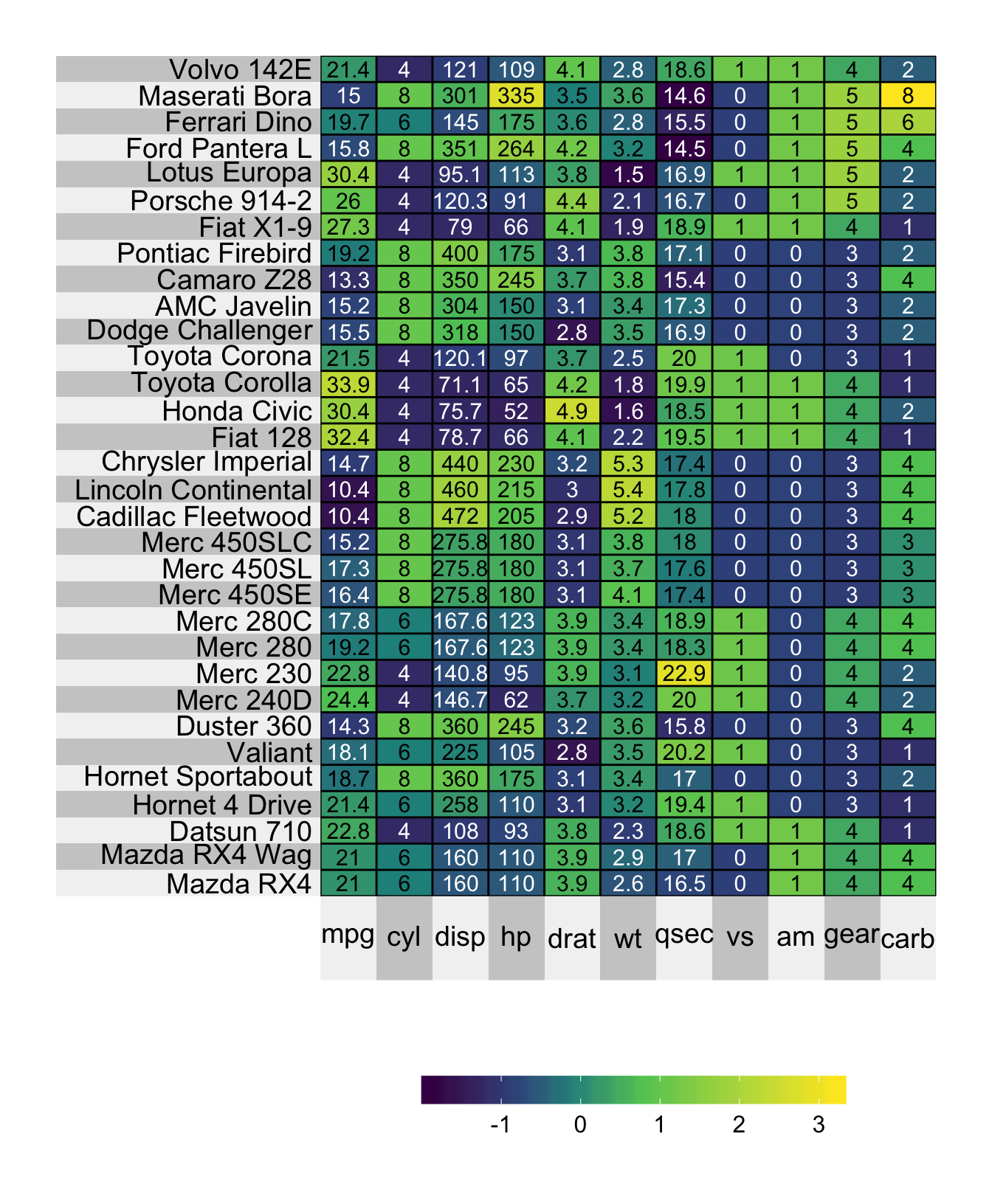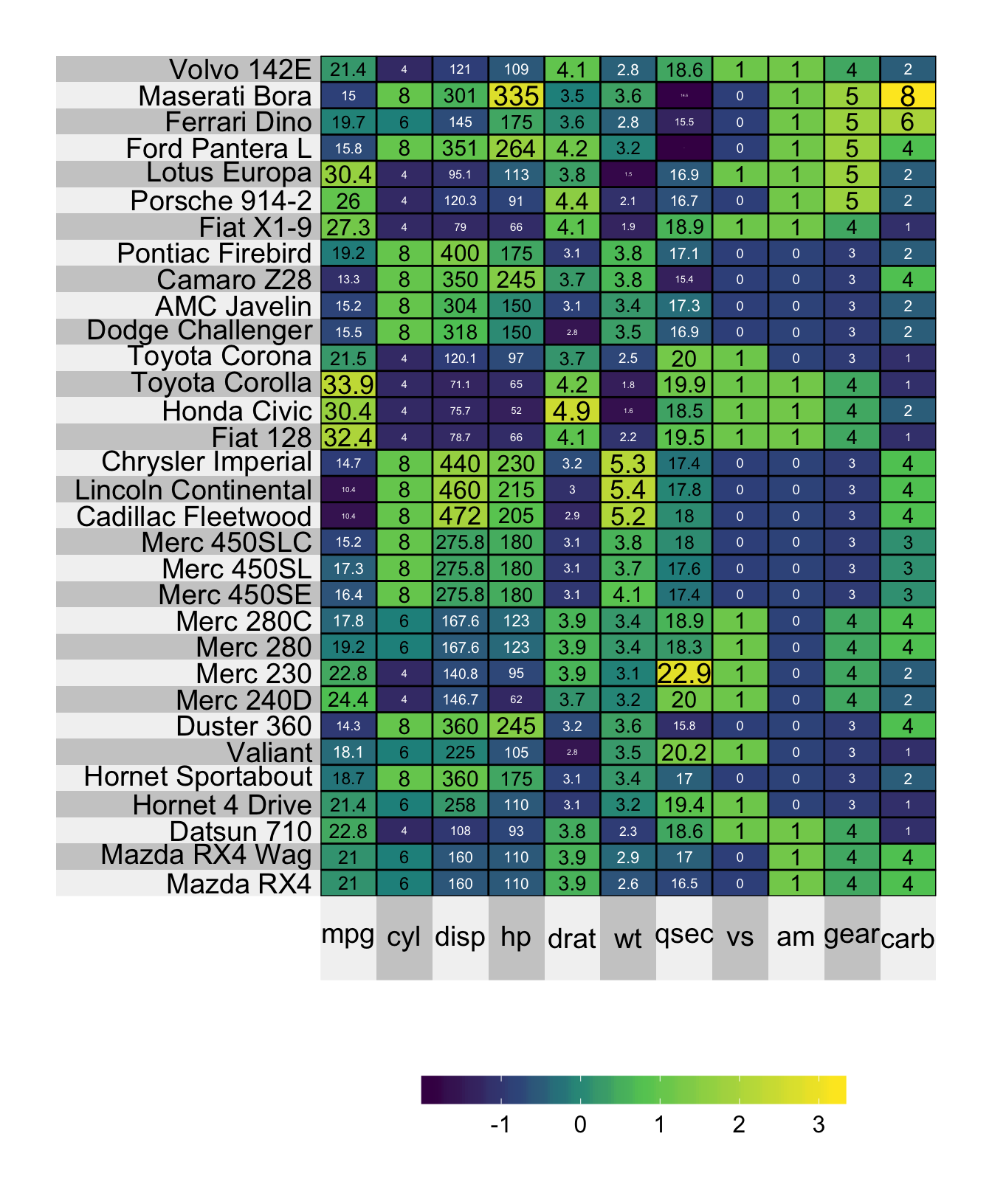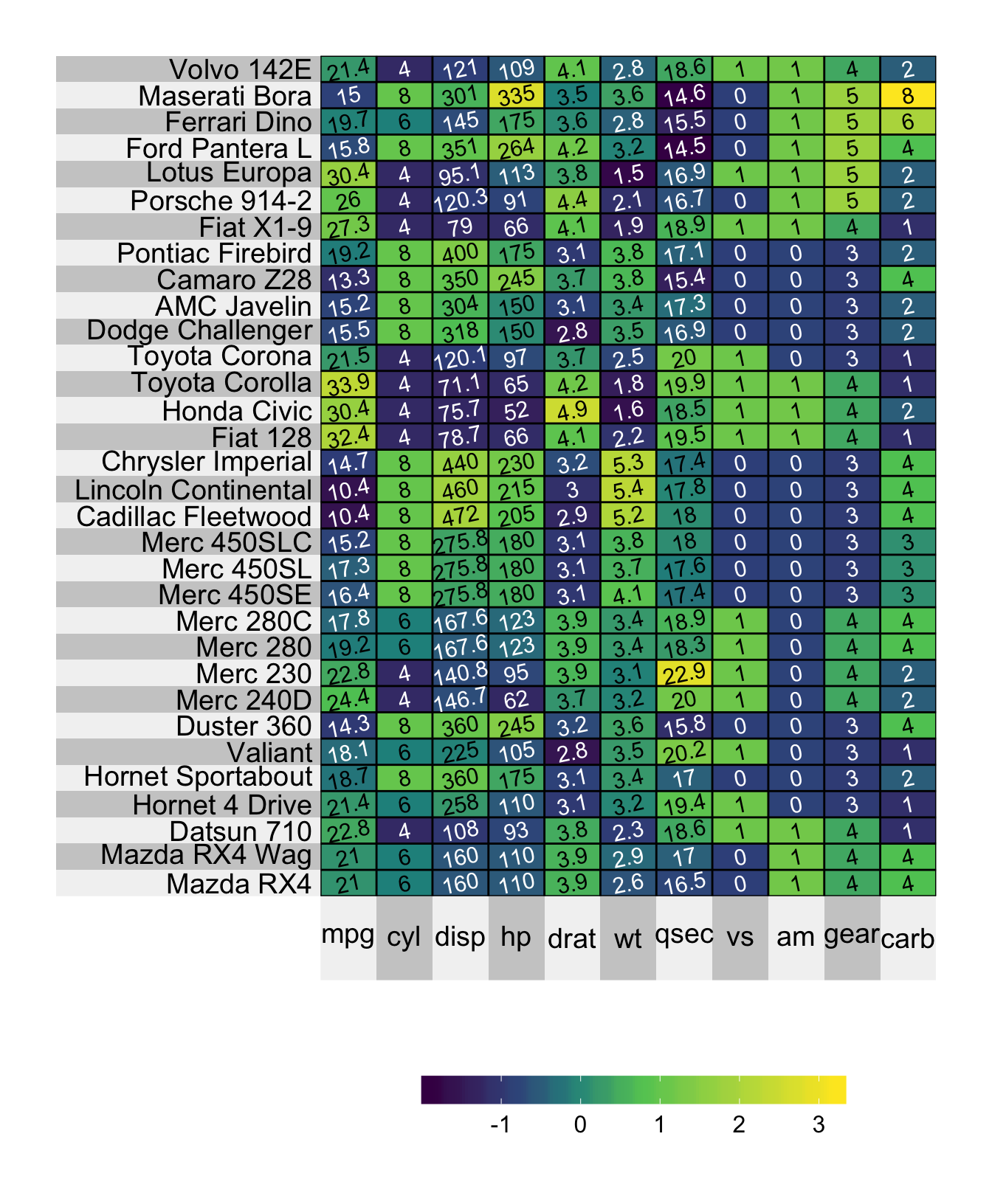It is easy to add text to each cell in the heatmap using the X.text argument. Below we simply plot the raw matrix over the top of the heatmap, but you could add any matrix of numbers or character strings.

superheat(X = mtcars, # heatmap matrix
# scale the matrix columns
scale = TRUE,
X.text = round(as.matrix(mtcars), 1),
X.text.size = 4)## 9.1 Text color

You can change the colors of the text by providing a single number or a matrix of numbers to the argument X.text.col. If providing a matrix, the dimension must be identical to that of the X matrix provided.

# set the text colors
# identify all scaled values that fall below -0.3
mtcars.col <- scale(mtcars) < -0.3
# set all values that satisfy the condition to "white"
mtcars.col <- gsub("TRUE", "white", mtcars.col)
# set all values that do not satisfy the condition to "black"
mtcars.col <- gsub("FALSE", "black", mtcars.col)
# convert to matrix
mtcars.col <- matrix(mtcars.col, ncol = ncol(mtcars))

superheat(X = mtcars, # heatmap matrix
# scale the matrix columns
scale = TRUE,
X.text = round(as.matrix(mtcars), 1),
X.text.col = mtcars.col,
X.text.size = 4)## 9.2 Font size

You can change size of the text by providing a single number or a matrix of numbers to the argument X.text.size. If providing a matrix, the dimension must be identical to that of the X matrix provided.

# Set the size to simply be proportional to the scaled value
mtcars.size <- scale(mtcars) + 2
superheat(X = mtcars, # heatmap matrix
# scale the matrix columns
scale = TRUE,
X.text = round(as.matrix(mtcars), 1),
X.text.col = mtcars.col,
X.text.size = mtcars.size)## 9.3 Text angle

You can change the angle of the text by providing a single number (the number of degrees between 0 and 360) or a matrix of numbers to the argument X.text.angle. If providing a matrix, the dimension must be identical to that of the X matrix provided.

superheat(X = mtcars, # heatmap matrix
# scale the matrix columns
scale = TRUE,
X.text.angle = 12)